Difference between revisions of "TIP4P/2005 model of water"

The TIP4P/2005 model  is a re-parameterisation of the original TIP4P potential for simulations of water. TIP4P/2005 is a rigid planar model, having a similar geometry to that of the Bernal and Fowler model.

Parameters

The TIP4P/2005 model consists of a Lennard-Jones site for the oxygen atom, and three charge sites.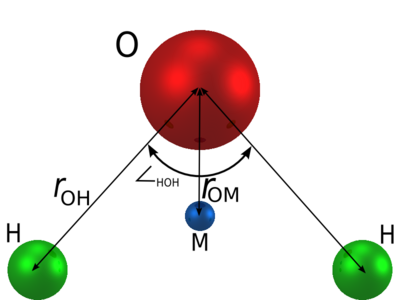$r_{\mathrm {OH}}$ (Å)$\angle$HOH , deg$\sigma$ (Å)$\epsilon/k$ (K) q(O) (e) q(H) (e) q(M) (e)$r_{\mathrm {OM}}$ (Å) 0.9572 104.52 3.1589 93.2 0 0.5564 -2q(H) 0.1546

Phase diagram

The phase diagram of the TIP4P/2005 model in the pressure-temperature plane (adapted from Fig. 9a of ) is given in a publication by Abascal, Sanz and Vega  (revisited by Conde et. al. ) and for negative pressures in the publication .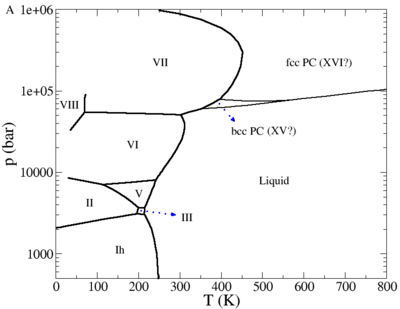Plastic crystal phases

Recent simulations have suggested the possibility of a plastic crystal phase or phases for water 

Supercooled region

The supercooled region has been studied by Abascal and Vega , locating a Widom line , indicating a second critical point for water located at 1350 bar and 193 K.

Surface tension

The surface tension has been studied for the TIP4P/2005 model, giving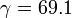$\gamma = 69.1$ mJ/m2 at 300 K using the test area method ,$\gamma = 68.4$ mN/m at 300 K using using the Ewald sums for the dispersion interactions ,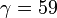$\gamma = 59$ mJ/m2 at 350 K using the reaction field method  and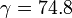$\gamma = 74.8$ mN/m at 249 K .

Self-diffusion coefficient

The TIP4P/2005 potential has a self-diffusion coefficient, in bulk water at 298 K, of 0.21 Å2 ps−1 in a classical simulation of 216 water molecules (experimental value: 0.23 Å2 ps−1) . See also .

Shear viscosity

The shear viscosity for the TIP4P/2005 model is 0.855 mPa.s at 298 K and 1 bar  (experimental value 0.896 mPa.s ).

Liquid-liquid critical point

For the TIP4P/2005 model the liquid-liquid critical point is located at: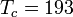$T_c = 193$K,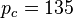$p_c =135$ MPa and having a density of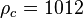$\rho_c =1012$ kg/m3 .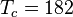$T_c = 182$K,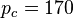$p_c =170$ MPa and having a density of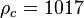$\rho_c =1017$ kg/m3 .

Structure factor

The structure factor, in particular for small wave vectors, has been calculated by Sedlmeier, Horinek and Netz , who observe that the TIP4P/2005 model yields an "almost quantitative agreement".

Virial coefficients

The second virial coefficient has been calculated by Chialvo et al .

Melting point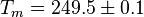$T_m = 249.5 \pm 0.1$ K .